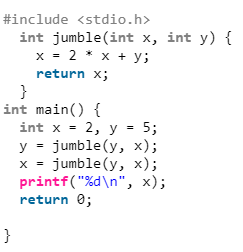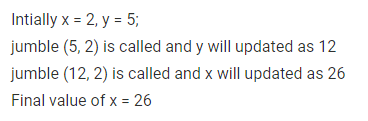# Gate CS-2019 Question Paper With Solutions

Q. 27 Consider the following C program:

``#include <stdio.h> ` `  ``int` `jumble(``int` `x, ``int` `y) { ` `    ``x = 2 * x + y; ` `    ``return` `x; ` `  ``} ` `int` `main() { ` `  ``int` `x = 2, y = 5; ` `  ``y = jumble(y, x); ` `  ``x = jumble(y, x); ` `  ``printf``(``"%d\n"``, x); ` `  ``return` `0; ` ` ` `}``

The value printed by program is __________ .

Note: This was Numerical Type question.

(A) 26

(B) 2

(C) 5

(D) 12